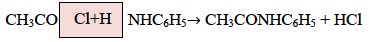# ISC Class 12 Chemistry Question Paper Solution 2016

The ISC Class 12 Chemistry – Paper I (Theory) was conducted on 11th March 2016. The paper was of 3 hours of time duration and 70 Marks. The exam started at 2 PM. The ISC Class 12 Chemistry Solved Question Paper 2016 is available here for students preparation. Students search for last year’s ISC Class 12 Chemistry Question Papers with answers but find it difficult to get. So, to help them, we have provided the ISC Class 12 Chemistry Question Paper Solution pdf 2016 from the link provided below. Access your answer sheet by comparing the answers provided in the solution pdf. Find out the mistakes and try not to repeat them in the future.

For students convenience, we have also compiled the solved ISC Class 12 Previous Year Question Papers of Maths, Physics, Chemistry and Biology subjects at one place. Students can download and access them for free. They can have a look at the ISC Class 12 Chemistry Question Paper Solution 2016 below.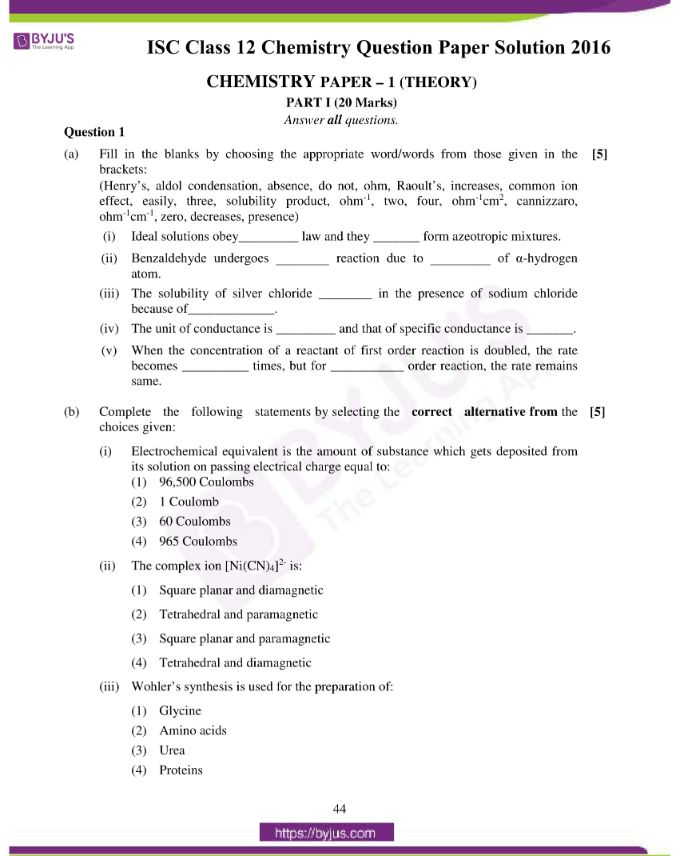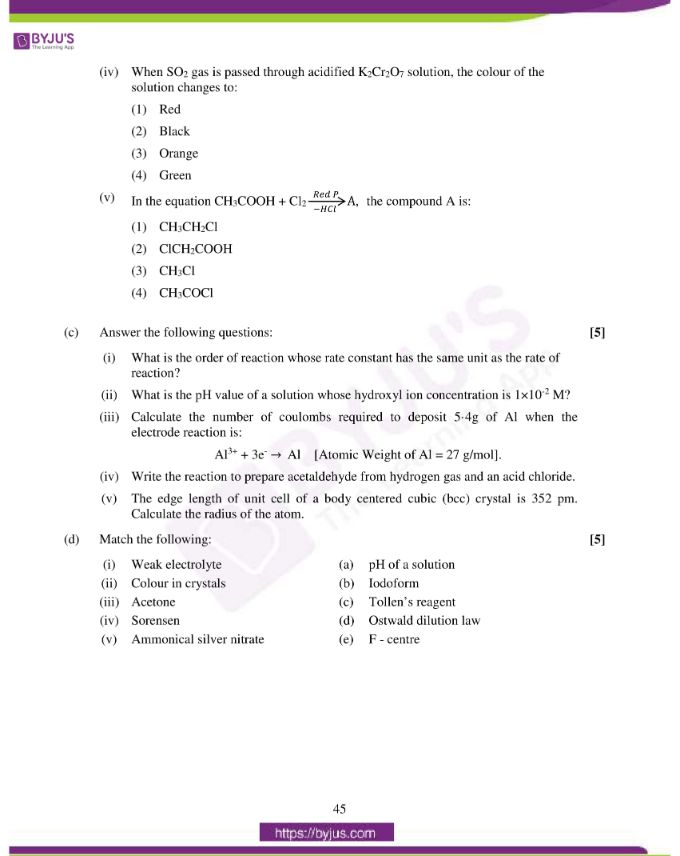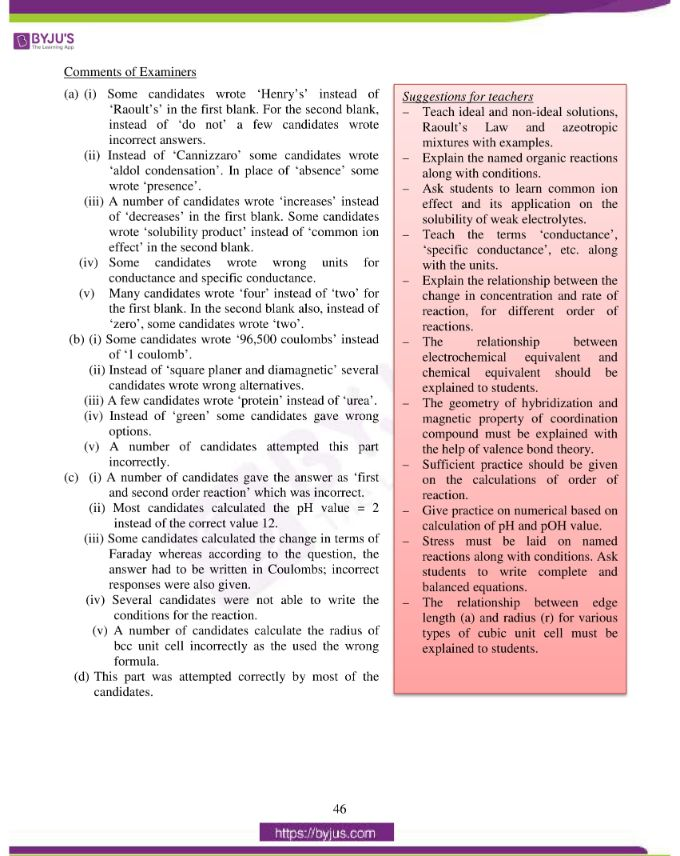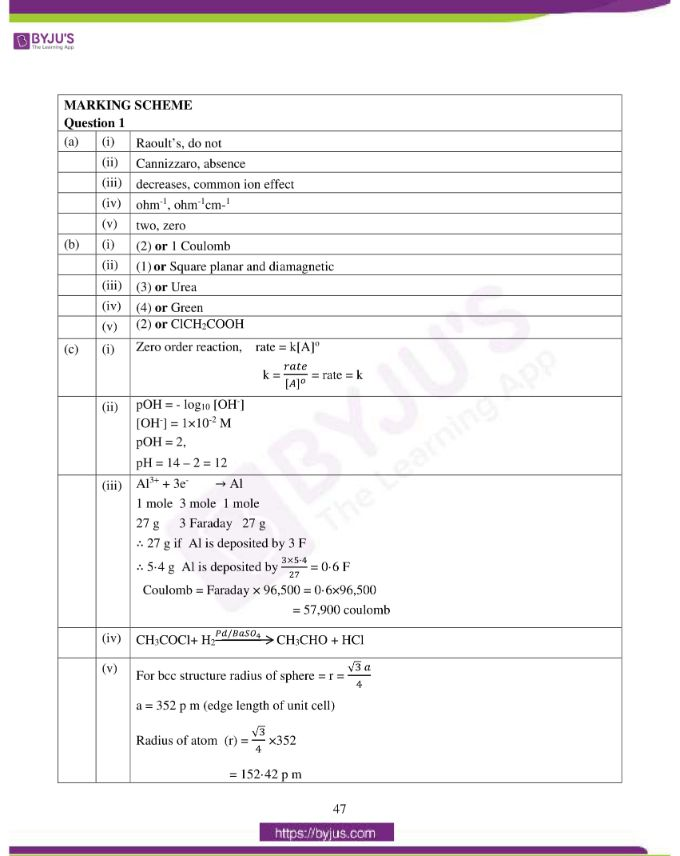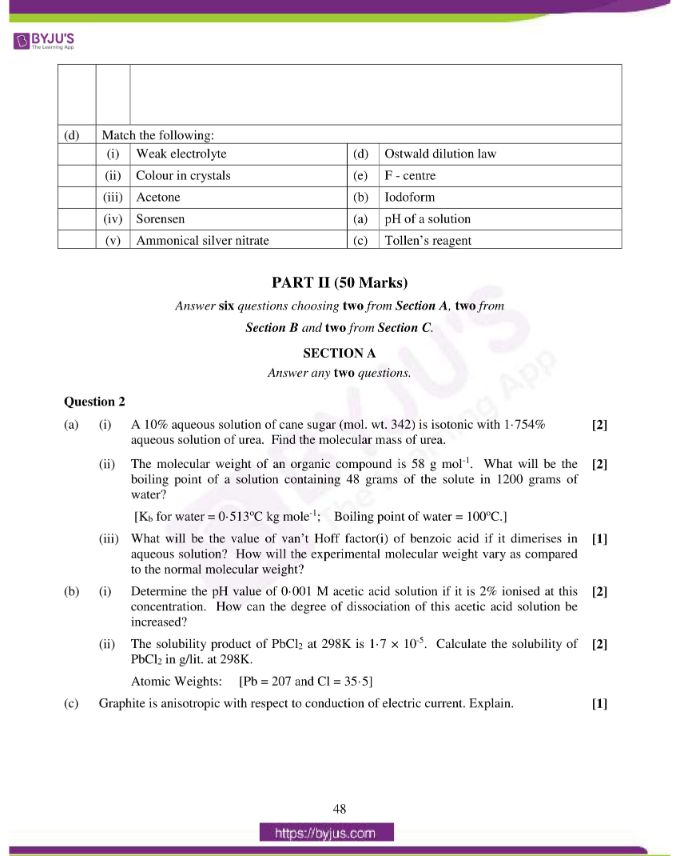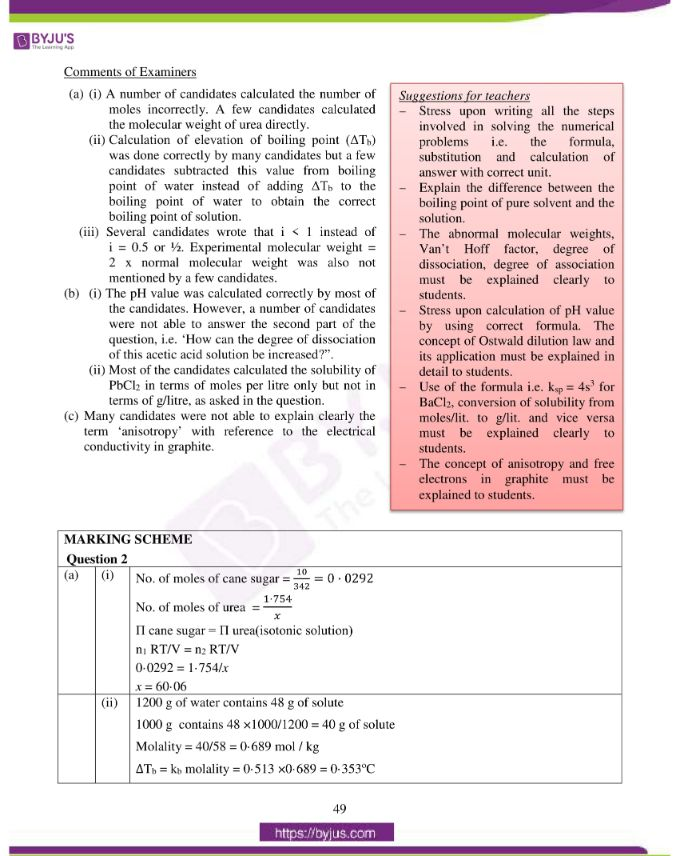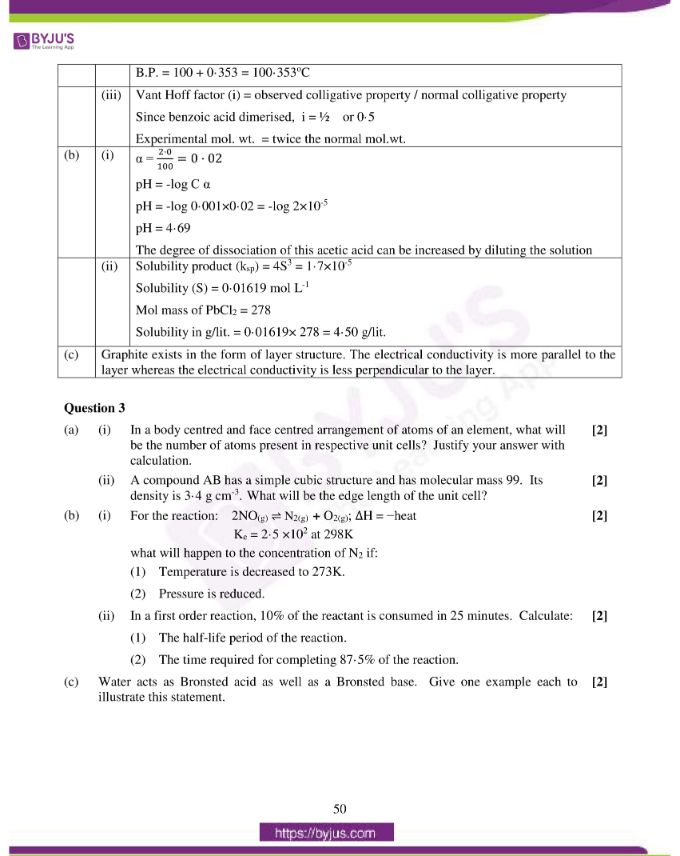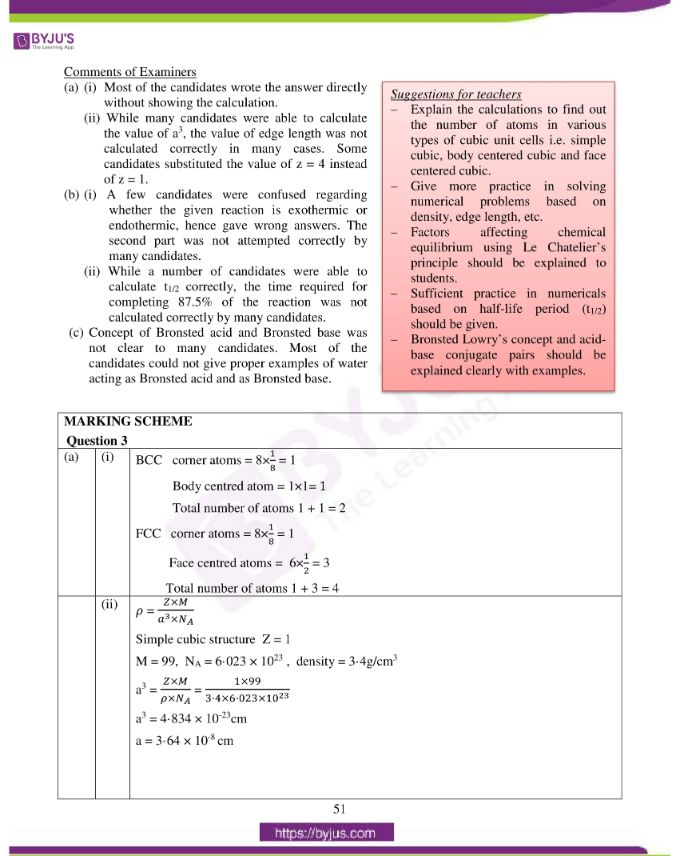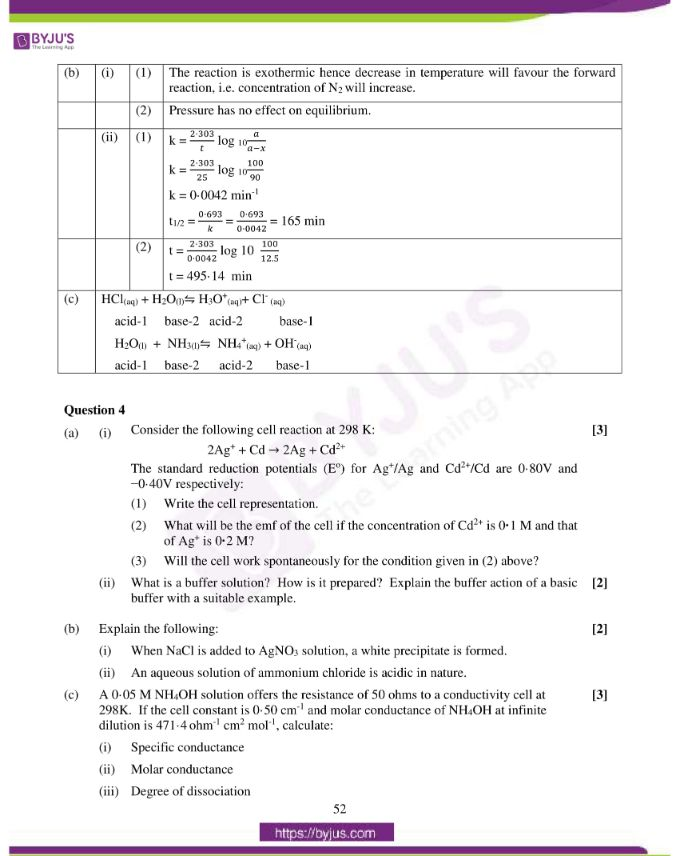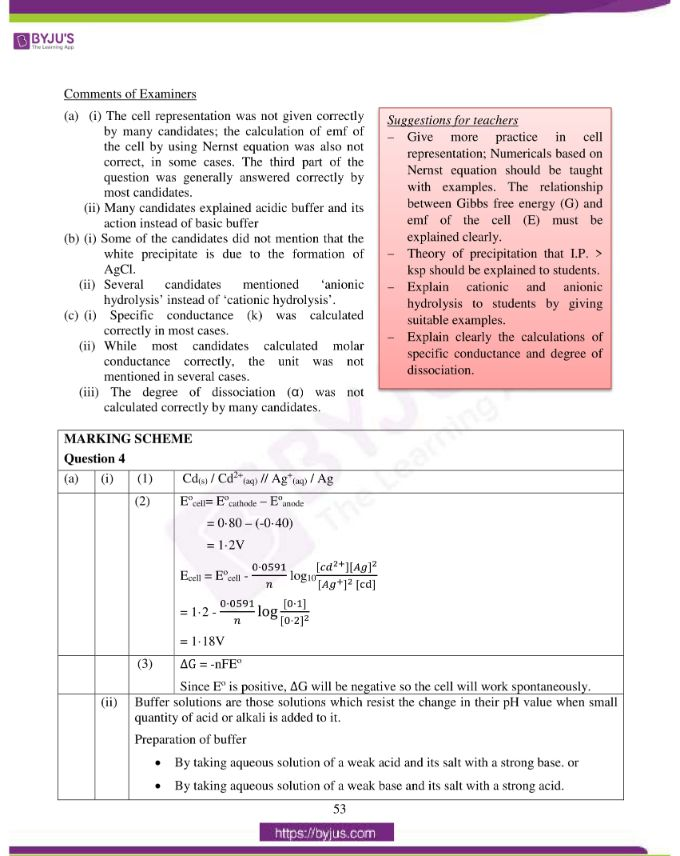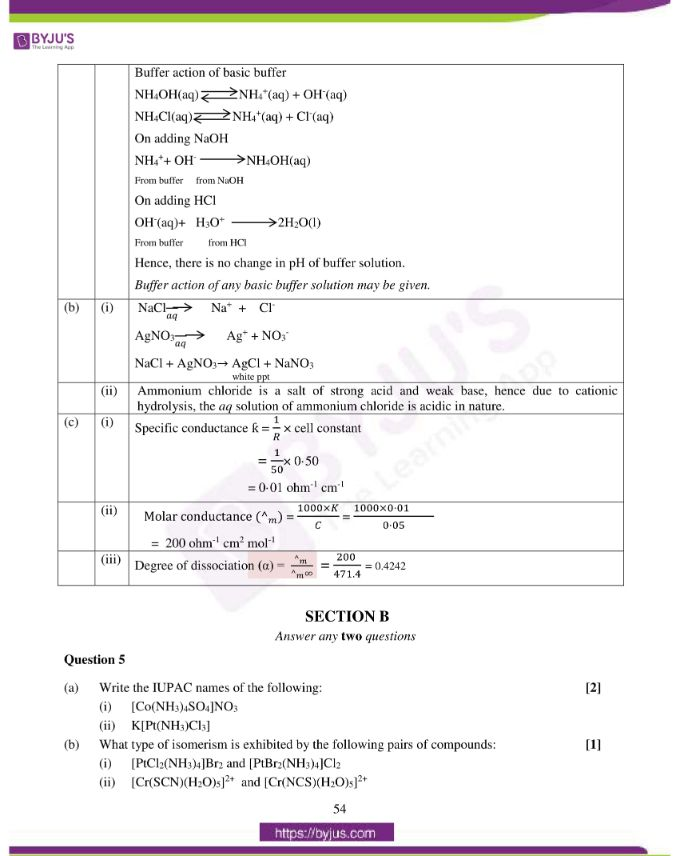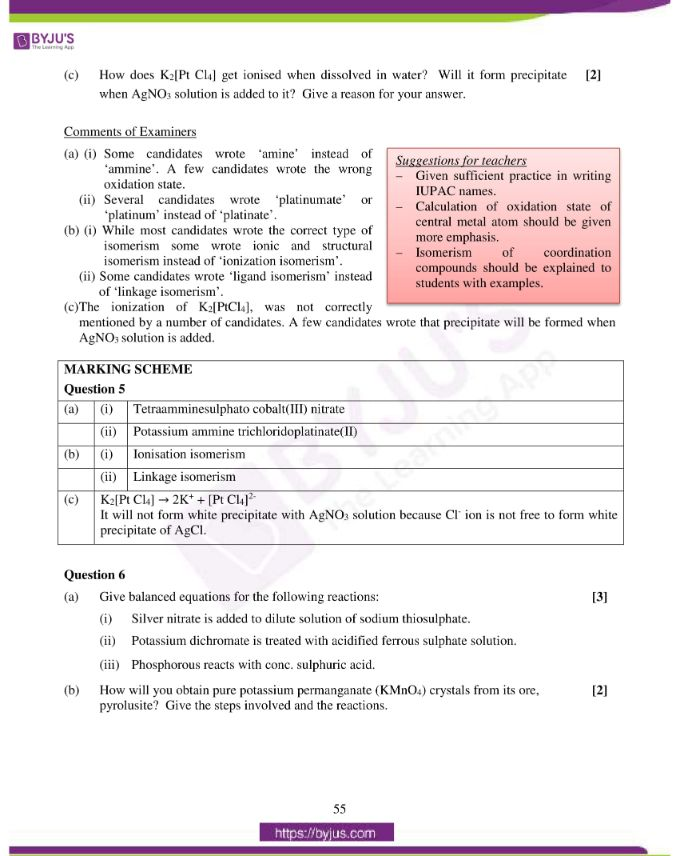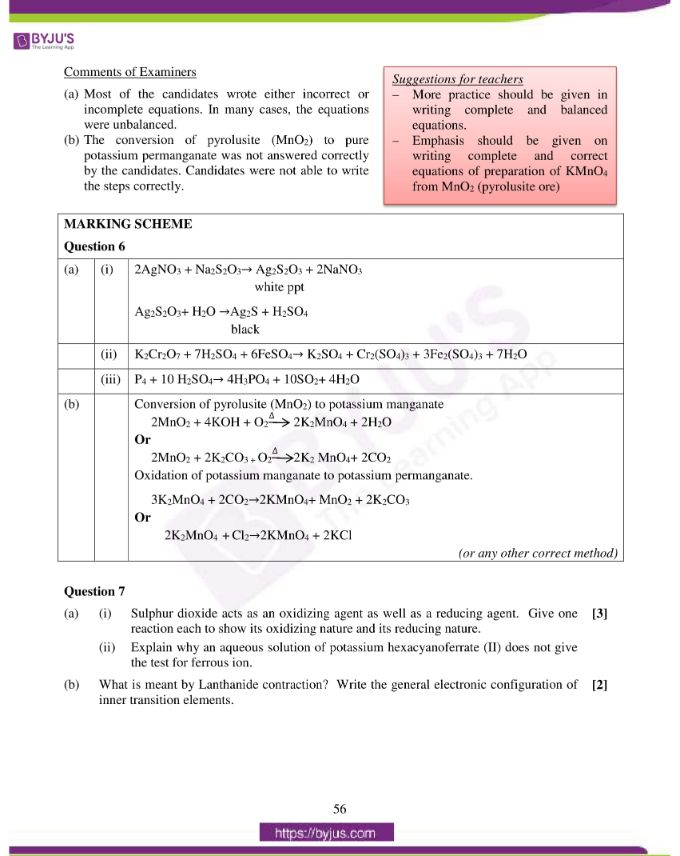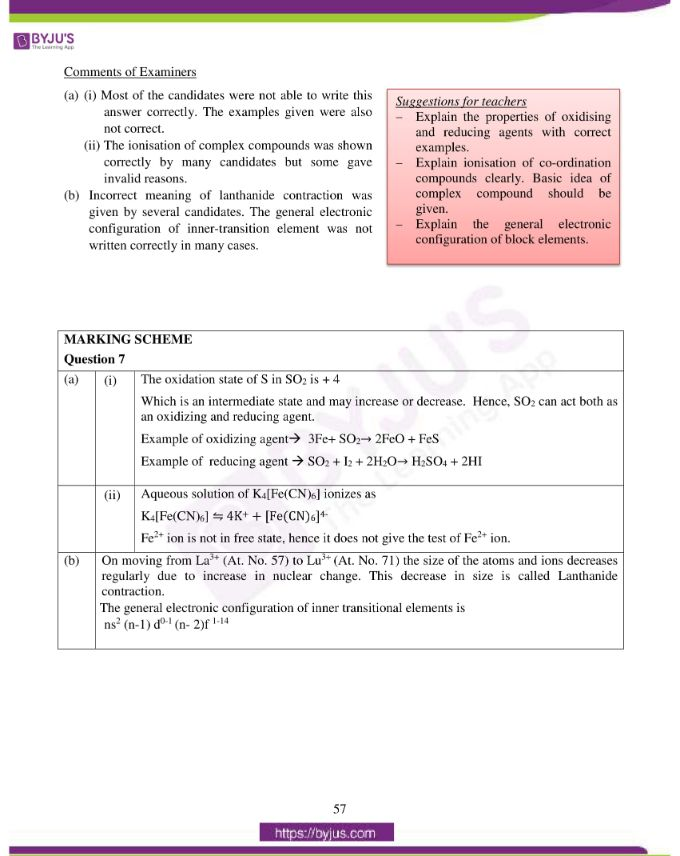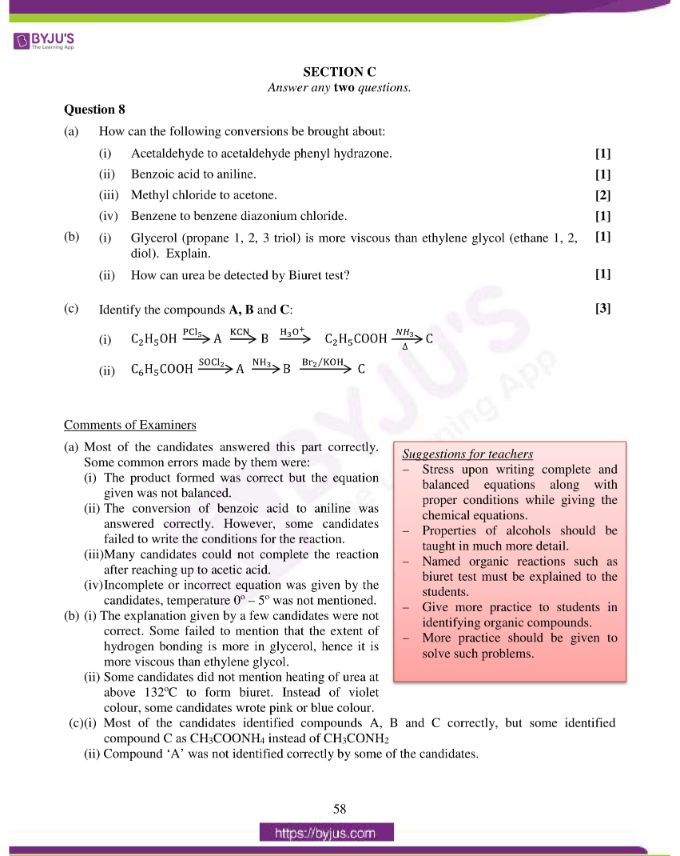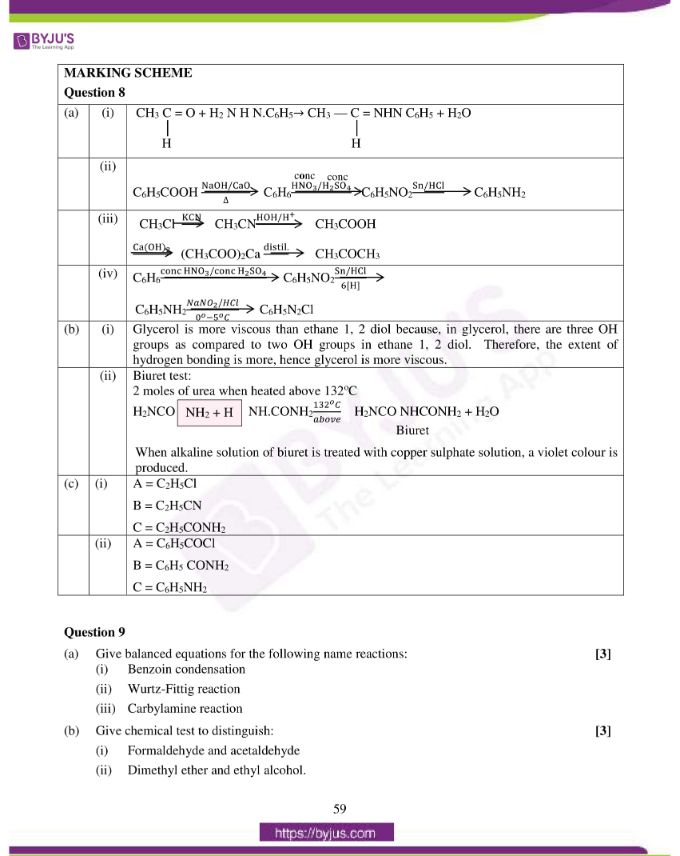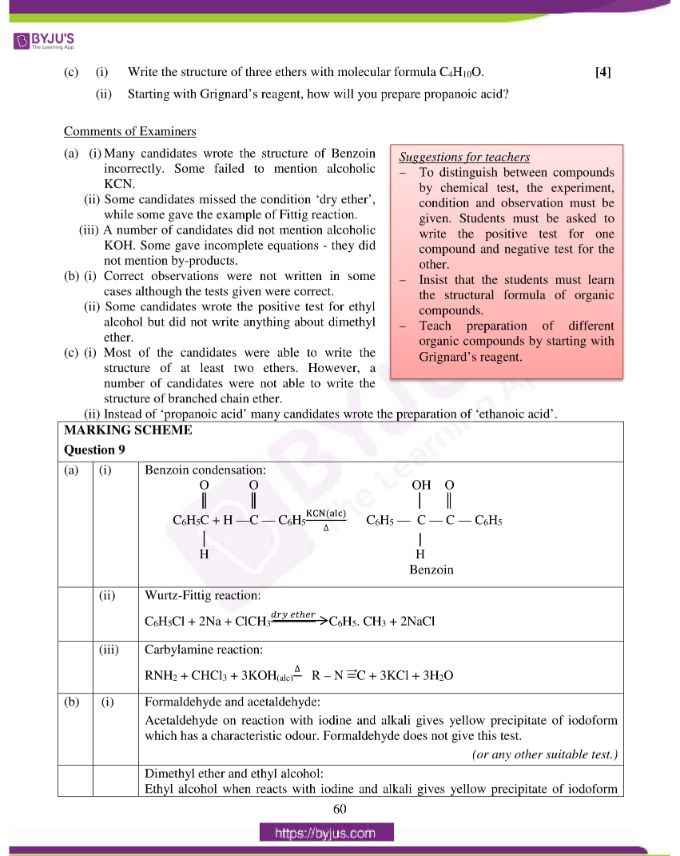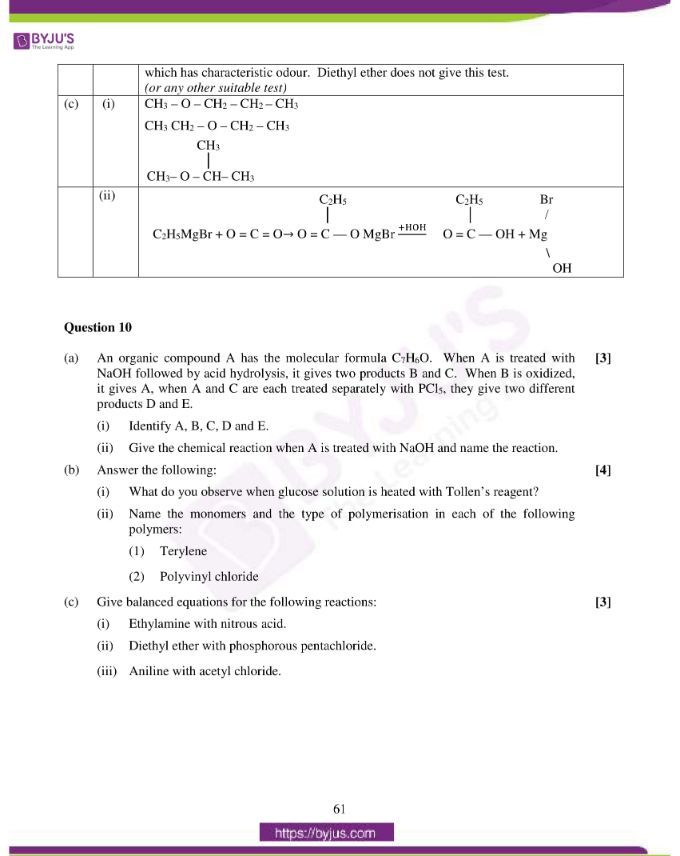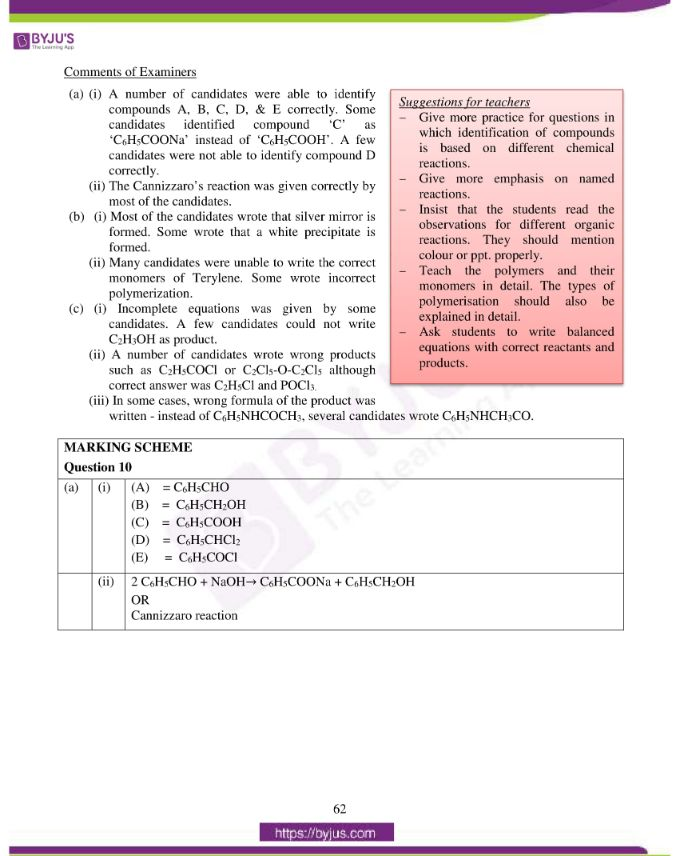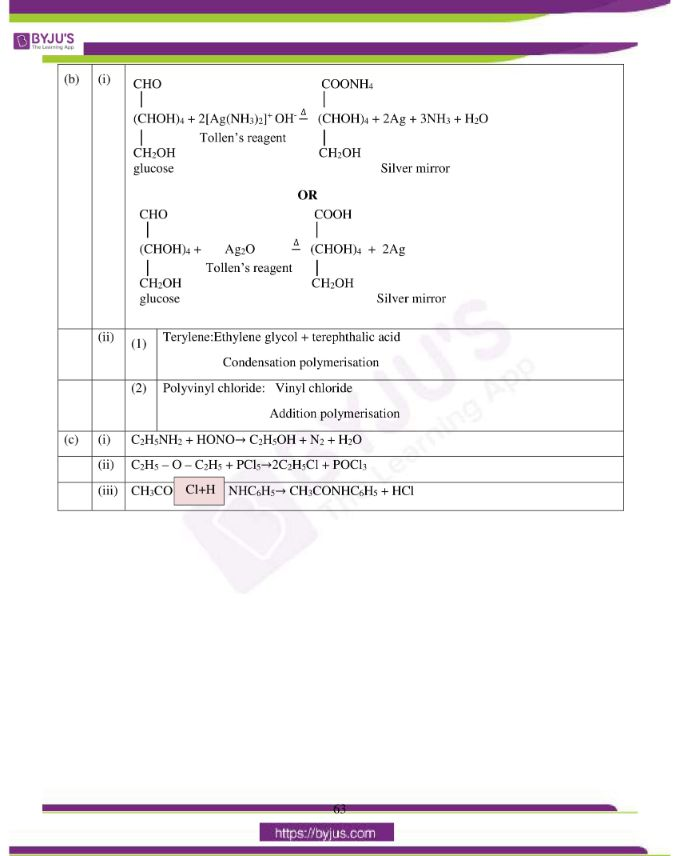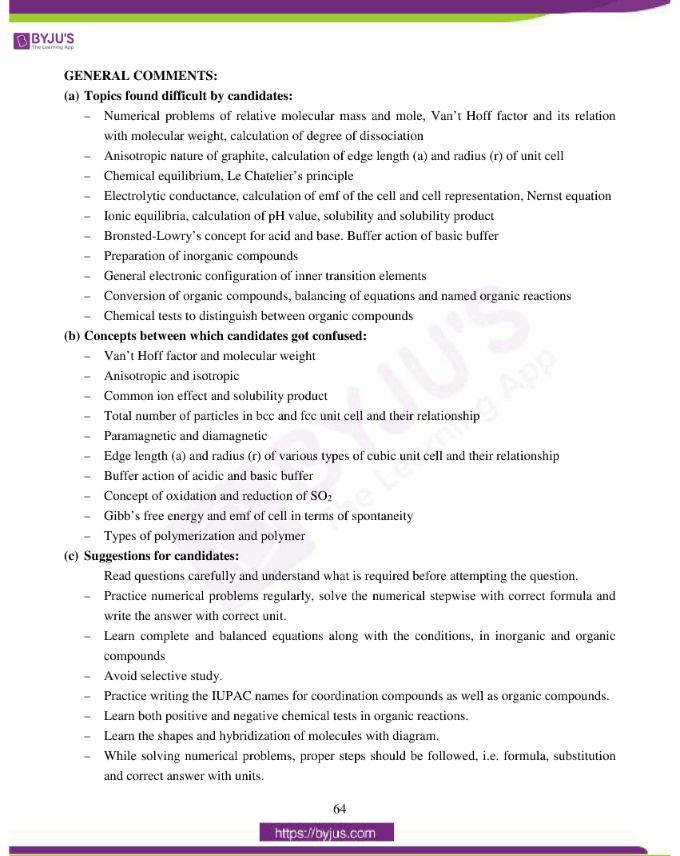### Difficult Topics of ISC Class 12 Chemistry Paper 2016

Topics which students found difficult while solving the Chemistry 2016 paper are mentioned below:

− Numerical problems of relative molecular mass and mole, Van’t Hoff factor and its relation with molecular weight, calculation of degree of dissociation.

− Anisotropic nature of graphite, calculation of edge length (a) and radius (r) of unit cell

− Chemical equilibrium, Le Chatelier’s principle.

− Electrolytic conductance, calculation of emf of the cell and cell representation, Nernst equation.

− Ionic equilibria, calculation of pH value, solubility and solubility product.

− Bronsted-Lowry’s concept for acid and base. Buffer action of basic buffer.

− Preparation of inorganic compounds.

− General electronic configuration of inner transition elements.

− Conversion of organic compounds, balancing of equations and named organic reactions.

− Chemical tests to distinguish between organic compounds.

### Confusing ISC Class 12 Chemistry Questions 2016

Chemistry concepts between which students got confused during the exam are mentioned below.

− Van’t Hoff factor and molecular weight

− Anisotropic and isotropic

− Common ion effect and solubility product

− Total number of particles in bcc and fcc unit cell and their relationship

− Paramagnetic and diamagnetic

− Edge length (a) and radius (r) of various types of cubic unit cell and their relationship

− Buffer action of acidic and basic buffer

− Concept of oxidation and reduction of SO2

− Gibb’s free energy and emf of cell in terms of spontaneity

− Types of polymerization and polymer

## ISC Class 12 Chemistry Question Paper Solution 2016

### Question 1:

(a) Fill in the blanks by choosing the appropriate word/words from those given in the brackets: (Henry’s, aldol condensation, absence, do not, ohm, Raoult’s, increases, common ion effect, easily, three, solubility product, ohm-1, two, four, ohm-1cm2, cannizzaro, ohm-1cm-1, zero, decreases, presence)

(i) Ideal solutions obey_________ law and they _______ form azeotropic mixtures.

(ii) Benzaldehyde undergoes ________ reaction due to _________ of α-hydrogen atom.

(iii) The solubility of silver chloride ________ in the presence of sodium chloride because of_____________.

(iv) The unit of conductance is _________ and that of specific conductance is _______.

(v) When the concentration of a reactant of first order reaction is doubled, the ratebecomes __________ times, but for ___________ order reaction, the rate remains the same.

(b) Complete the following statements by selecting the correct alternative from the choices given:

(i) Electrochemical equivalent is the amount of substance which gets deposited from its solution on passing electrical charge equal to:

1. 96,500 Coulombs
2. 1 Coulomb
3. 60 Coulombs
4. 965 Coulombs

(ii) The complex ion [Ni(CN)4]2- is:

1. Square planar and diamagnetic
2. Tetrahedral and paramagnetic
3. Square planar and paramagnetic
4. Tetrahedral and diamagnetic

(iii) Wohler’s synthesis is used for the preparation of:

1. Glycine
2. Amino acids
3. Urea
4. Proteins

(iv) When SO2 gas is passed through acidified K2Cr2O7 solution, the colour of the solution changes to:

1. Red
2. Black
3. Orange
4. Green

(v) In the equation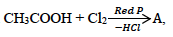the compound A is:

1. CH3CH2Cl
2. ClCH2COOH
3. CH3Cl
4. CH3COCl

(i) What is the order of reaction whose rate constant has the same unit as the rate of reaction?

(ii) What is the pH value of a solution whose hydroxyl ion concentration is 1×10-2 M?

(iii) Calculate the number of coulombs required to deposit 5·4g of Al when the electrode reaction is:

Al3+ + 3e → Al [Atomic Weight of Al = 27 g/mol].

(iv) Write the reaction to prepare acetaldehyde from hydrogen gas and an acid chloride.

(v) The edge length of the unit cell of a body centered cubic (bcc) crystal is 352 pm.Calculate the radius of the atom.

(d) Match the following:

 (i) Weak electrolyte (a) pH of a solution (ii) Colour in crystals (b) Iodoform (iii) Acetone (c) Tollen’s reagent (iv) Sorensen (d) Ostwald dilution law (v) Ammonical silver nitrate (e) F – centre

(a) (i) Raoult’s, do not

(ii) Cannizzaro, absence

(iii) decreases, common ion effect

(iv) ohm-1, ohm-1cm-1

(v) two, zero

(b) (i) (2)or 1 Coulomb

(ii) (1)or Square planar and diamagnetic

(iii) (3)or Urea

(iv) (4)or Green

(v) (2)or ClCH2COOH

(c) (i) Zero order reaction, rate = k[A]o

k = rate /[A]o = rate = k

(ii) pOH = – log10 [OH] [OH] = 1×10-2 M

pOH = 2,

pH = 14 – 2 = 12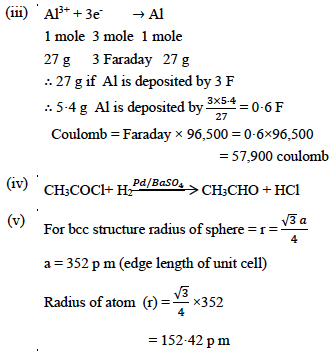(d)

 (i) Weak electrolyte (d) Ostwald dilution law (ii) Colour in crystals (e) F – centre (iii) Acetone (b) Iodoform (iv) Sorensen (a) pH of a solution (v) Ammonical silver nitrate (c) Tollen’s reagent

### Question 2:

(a) (i) A 10% aqueous solution of cane sugar (mol. wt. 342) is isotonic with 1·754%aqueous solution of urea. Find the molecular mass of urea.

(ii) The molecular weight of an organic compound is 58 g mol-1. What will be the boiling point of a solution containing 48 grams of the solute in 1200 grams of water? [Kb for water = 0·513oC kg mol-1; Boiling point of water = 100oC.]

(iii) What will be the value of the van’t Hoff factor(i) of benzoic acid if it diminishes in aqueous solution? How will the experimental molecular weight vary as compared to the normal molecular weight?

(b) (i) Determine the pH value of 0·001 M acetic acid solution if it is 2% ionised at this concentration. How can the degree of dissociation of this acetic acid solution be increased?

(ii) The solubility product of PbCl2 at 298K is 1·7 × 10-5. Calculate the solubility of PbCl2 in g/lit. at 298K.

Atomic Weights: [Pb = 207 and Cl = 35·5]

(c) Graphite is anisotropic with respect to conduction of electric current. Explain.

Answer: (a) (i) No. of moles of cane sugar = 10/342=0·0292

No. of moles of urea = 1·754/𝑥

Π cane sugar = Π urea (isotonic solution)

n1 RT/V = n2RT/V

0·0292 = 1·754/x

x = 60·06

(ii) 1200 g of water contains 48 g of solute

1000 g contains 48 ×1000/1200 = 40 g of solute

Molality = 40/58 = 0·689 mol / kg

ΔTb = kb molality = 0·513 ×0·689 = 0·353oC

B.P. = 100 + 0·353 = 100·353oC

(iii) Van’t Hoff factor (i) = observed colligative property / normal colligative property

Since benzoic acid dimerised, i = ½ or 0·5

Experimental mol. wt. = twice the normal mol.wt.

(b) (i) α = 2·0/100=0·02

pH = -log C α

pH = -log 0·001×0·02 = -log 2×10-5

pH = 4·69

The degree of dissociation of this acetic acid can be increased by diluting the solution

(ii) Solubility product (ksp) = 4S3 = 1·7×10-5

Solubility (S) = 0·01619 mol L-1

Mol mass of PbCl2 = 278

Solubility in g/lit. = 0·01619× 278 = 4·50 g/lit.

(c) Graphite exists in the form of layer structure. The electrical conductivity is more parallel to the layer whereas the electrical conductivity is less perpendicular to the layer.

### Question 3:

(a) (i) In a body centred and face centred arrangement of atoms of an element, what will be the number of atoms present in respective unit cells? Justify your answer with calculation.

(ii) A compound AB has a simple cubic structure and has molecular mass 99. Its Density is 3·4 g cm-3. What will be the edge length of the unit cell?

(b) (i) For the reaction: 2NO(g) ⇌ N2(g) + O2(g); ΔH = −heat

Ke = 2·5 ×102 at 298K

what will happen to the concentration of N2 if:

1. Temperature is decreased to 273K.
2. Pressure is reduced.

(ii) In a first order reaction, 10% of the reactant is consumed in 25 minutes. Calculate:

1. The half-life period of the reaction.
2. The time required for completing 87·5% of the reaction.

(c) Water acts as Bronsted acid as well as a Bronsted base. Give one example each to illustrate this statement.

Answer: (a) (i) BCC corner atoms = 8×1/8 = 1

Body centred atom = 1×1= 1

Total number of atoms 1 + 1 = 2

FCC corner atoms = 8×1/8 = 1

Face centred atoms = 6×1/2 = 3

Total number of atoms 1 + 3 = 4

(ii)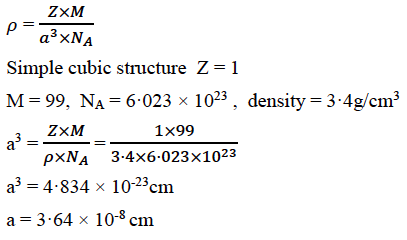(b) (i)

1. The reaction is exothermic hence decrease in temperature will favour the forward reaction, i.e. concentration of N2 will increase.
2. Pressure has no effect on equilibrium.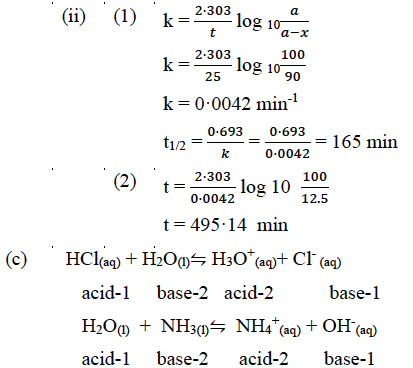### Question 4:

(a) (i) Consider the following cell reaction at 298 K:

2Ag+ + Cd → 2Ag + Cd2+

The standard reduction potentials (Eo) for Ag+/Ag and Cd2+/Cd are 0·80V and −0·40V respectively:

1. Write the cell representation.
2. What will be the emf of the cell if the concentration of Cd2+ is 0·1 M and that of Ag+ is 0·2 M?
3. Will the cell work spontaneously for the condition given in (2) above?

(ii) What is a buffer solution? How is it prepared? Explain the buffer action of a basic buffer with a suitable example.

(b) Explain the following:

(i) When NaCl is added to AgNO3 solution, a white precipitate is formed.

(ii) An aqueous solution of ammonium chloride is acidic in nature.

(c) A 0·05 M NH4OH solution offers the resistance of 50 ohms to a conductivity cell at 298 K. If the cell constant is 0·50 cm-1 and molar conductance of NH4OH at infinite dilution is 471·4 ohm-1 cm2 mol-1, calculate:

(i) Specific conductance

(ii) Molar conductance

(iii) Degree of dissociation

1. Cd(s) / Cd2+(aq) // Ag+(aq) / Ag
2. Eocell = Eocathode – Eoanode

= 0·80 – (-0·40)

= 1·2V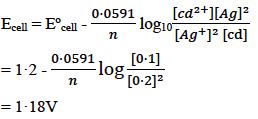1. ΔG = -nFEo

Since Eo is positive, ΔG will be negative so the cell will work spontaneously.

(ii) Buffer solutions are those solutions which resist the change in their pH value when a small quantity of acid or alkali is added to it.

Preparation of buffer

• By taking aqueous solution of a weak acid and its salt with a strong base. or
• By taking aqueous solution of a weak base and its salt with a strong acid.

Buffer action of basic buffer

NH4OH(aq) ⇌ NH4+(aq) + OH(aq)

NH4Cl(aq) ⇌ NH4+(aq) + Cl(aq)

NH4+ (from buffer) + OH (from NaOH) → NH4OH(aq)

OH(aq) (from buffer) + H3O+ (from HCl) → 2H2O(l)

Hence, there is no change in pH of buffer solution.

Buffer action of any basic buffer solution may be given.

(b) (i)

NaCl(aq) → Na+ + Cl

AgNO3(aq) → Ag+ + NO3

NaCl + AgNO3 → AgCl (white ppt) + NaNO3

(ii) Ammonium chloride is a salt of strong acid and weak base, hence due to cationic hydrolysis, the aq solution of ammonium chloride is acidic in nature.

(c) (i) Specific conductance k = 1/𝑅 × cell constant

= 1/50 × 0·50

= 0·01 ohm-1 cm-1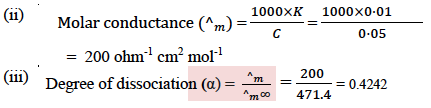### Question 5:

(a) Write the IUPAC names of the following:

(i) [Co(NH3)4SO4]NO3

(ii) K[Pt(NH3)Cl3]

(b) What type of isomerism is exhibited by the following pairs of compounds:

(i) [PtCl2(NH3)4]Br2 and [PtBr2(NH3)4]Cl2

(ii) [Cr(SCN)(H2O)5]2+ and [Cr(NCS)(H2O)5]2+

(c) How does K2[Pt Cl4] get ionised when dissolved in water? Will it form precipitate when an AgNO3 solution is added to it? Give a reason for your answer.

Answer: (a) (i) Tetraamminesulphato cobalt(III) nitrate

(ii) Potassium ammine trichloridoplatinate(II)

(b) (i) Ionisation isomerism

(c) K2[Pt Cl4] → 2K+ + [Pt Cl4]2-

It will not form white precipitate with AgNO3 solution because Cl ion is not free to form white precipitate of AgCl.

### Question 6:

(a) Give balanced equations for the following reactions:

(i) Silver nitrate is added to dilute solution of sodium thiosulphate.

(ii) Potassium dichromate is treated with acidified ferrous sulphate solution.

(iii) Phosphorous reacts with conc. sulphuric acid.

(b) How will you obtain pure potassium permanganate (KMnO4) crystals from its ore, pyrolusite? Give the steps involved and the reactions.

Answer: (a) (i) 2AgNO3 + Na2S2O3 → Ag2S2O3 (white ppt) + 2NaNO3

Ag2S2O3+ H2O → Ag2S (black) + H2SO4

(ii) K2Cr2O7 + 7H2SO4 + 6FeSO4 → K2SO4 + Cr2(SO4)3 + 3Fe2(SO4)3 + 7H2O

(iii) P4 + 10 H2SO4 → 4H3PO4 + 10SO2 + 4H2O

(b) Conversion of pyrolusite (MnO2) to potassium manganate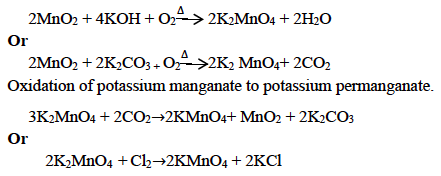### Question 7:

(a) (i) Sulphur dioxide acts as an oxidizing agent as well as a reducing agent. Give one reaction each to show its oxidizing nature and its reducing nature.

(ii) Explain why an aqueous solution of potassium hexacyanoferrate (II) does not give the test for ferrous ions.

(b) What is meant by Lanthanide contraction? Write the general electronic configuration of inner transition elements.

Answer: (a) (i) The oxidation state of S in SO2 is + 4

Which is an intermediate state and may increase or decrease. Hence, SO2 can act both as an oxidizing and reducing agent.

Example of oxidizing agent: 3Fe+ SO2 → 2FeO + FeS

Example of reducing agent: SO2 + I2 + 2H2O → H2SO4 + 2HI

(ii) Aqueous solution of K4[Fe(CN)6] ionizes as

K4[Fe(CN)6] ⇋ 4K+ + [Fe(CN)6]4-

Fe2+ ion is not in free state, hence it does not give the test of Fe2+ ion.

(b) On moving from La3+ (At. No. 57) to Lu3+ (At. No. 71) the size of the atoms and ions decreases regularly due to increase in nuclear change. This decrease in size is called Lanthanide contraction.

The general electronic configuration of inner transitional elements is

ns2 (n-1) d0-1 (n- 2)f1-14

### Question 8:

(a) How can the following conversions be brought about:

(i) Acetaldehyde to acetaldehyde phenyl hydrazone.

(ii) Benzoic acid to aniline.

(iii) Methyl chloride to acetone.

(iv) Benzene to benzene diazonium chloride.

(b) (i) Glycerol (propane 1, 2, 3 triol) is more viscous than ethylene glycol (ethane 1, 2,diol). Explain.

(ii) How can urea be detected by Biuret test?

(c) Identify the compounds A, B and C: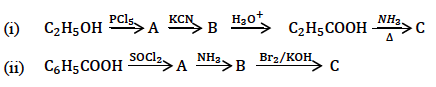(a)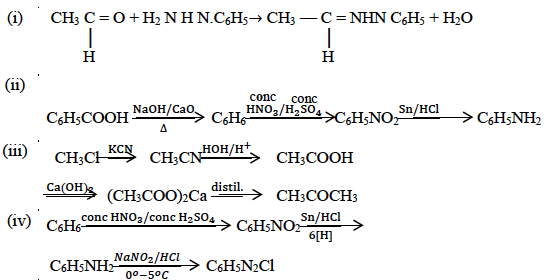(b) (i) Glycerol is more viscous than ethane 1, 2 diol because, in glycerol, there are three OH groups as compared to two OH groups in ethane 1, 2 diol. Therefore, the extent of hydrogen bonding is more, hence glycerol is more viscous.

(ii) Biuret test:

2 moles of urea when heated above 132oC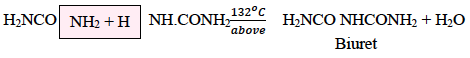When alkaline solution of biuret is treated with copper sulphate solution, a violet colour is produced.

(c) (i)

A = C2H5Cl

B = C2H5CN

C = C2H5CONH2

(ii) A = C6H5COCl

B = C6H5 CONH2

C = C6H5NH2

### Question 9:

(a) Give balanced equations for the following name reactions:

(i) Benzoin condensation

(ii) Wurtz-Fittig reaction

(iii) Carbylamine reaction

(b) Give chemical test to distinguish:

(i) Formaldehyde and acetaldehyde

(ii) Dimethyl ether and ethyl alcohol.

(c) (i) Write the structure of three ethers with molecular formula C4H10O.

(ii) Starting with Grignard’s reagent, how will you prepare propanoic acid?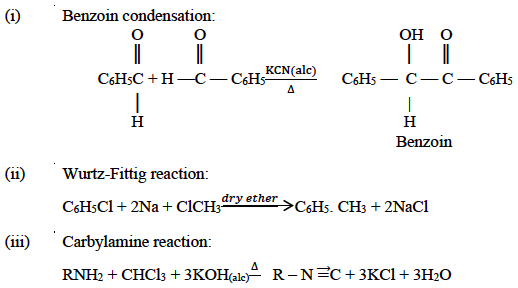(b) (i) Formaldehyde and acetaldehyde:

Acetaldehyde on reaction with iodine and alkali gives yellow precipitate of iodoform which has a characteristic odour. Formaldehyde does not give this test.

Dimethyl ether and ethyl alcohol:

Ethyl alcohol when reacts with iodine and alkali gives yellow precipitate of iodoform which has characteristic odour. Diethyl ether does not give this test.

(c)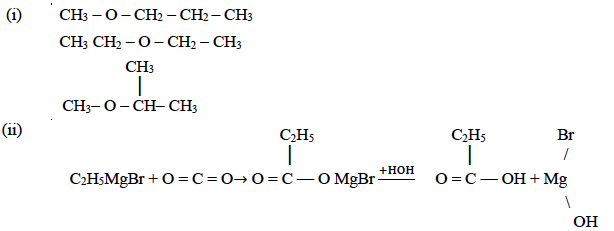### Question 10:

(a) An organic compound A has the molecular formula C7H6O. When A is treated with NaOH followed by acid hydrolysis, it gives two products B and C. When B is oxidized, it gives A, when A and C are each treated separately with PCl5, they give two different products D and E.

(i) Identify A, B, C, D and E.

(ii) Give the chemical reaction when A is treated with NaOH and name the reaction.

(i) What do you observe when glucose solution is heated with Tollen’s reagent?

(ii) Name the monomers and the type of polymerisation in each of the following polymers:

1. Terylene
2. Polyvinyl chloride

(c) Give balanced equations for the following reactions:

(i) Ethylamine with nitrous acid.

(ii) Diethyl ether with phosphorous pentachloride.

(iii) Aniline with acetyl chloride.

(a) (i) (A) = C6H5CHO

(B) = C6H5CH2OH

(C) = C6H5COOH

(D) = C6H5CHCl2

(E) = C6H5COCl

(ii) 2 C6H5CHO + NaOH → C6H5COONa + C6H5CH2OH

OR

Cannizzaro reaction

(b) (i)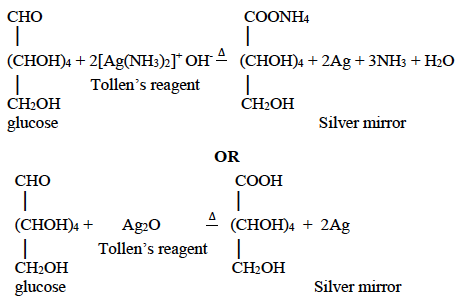(ii)

1. Terylene: Ethylene glycol + terephthalic acid

Condensation polymerisation

1. Polyvinyl chloride: Vinyl chloride